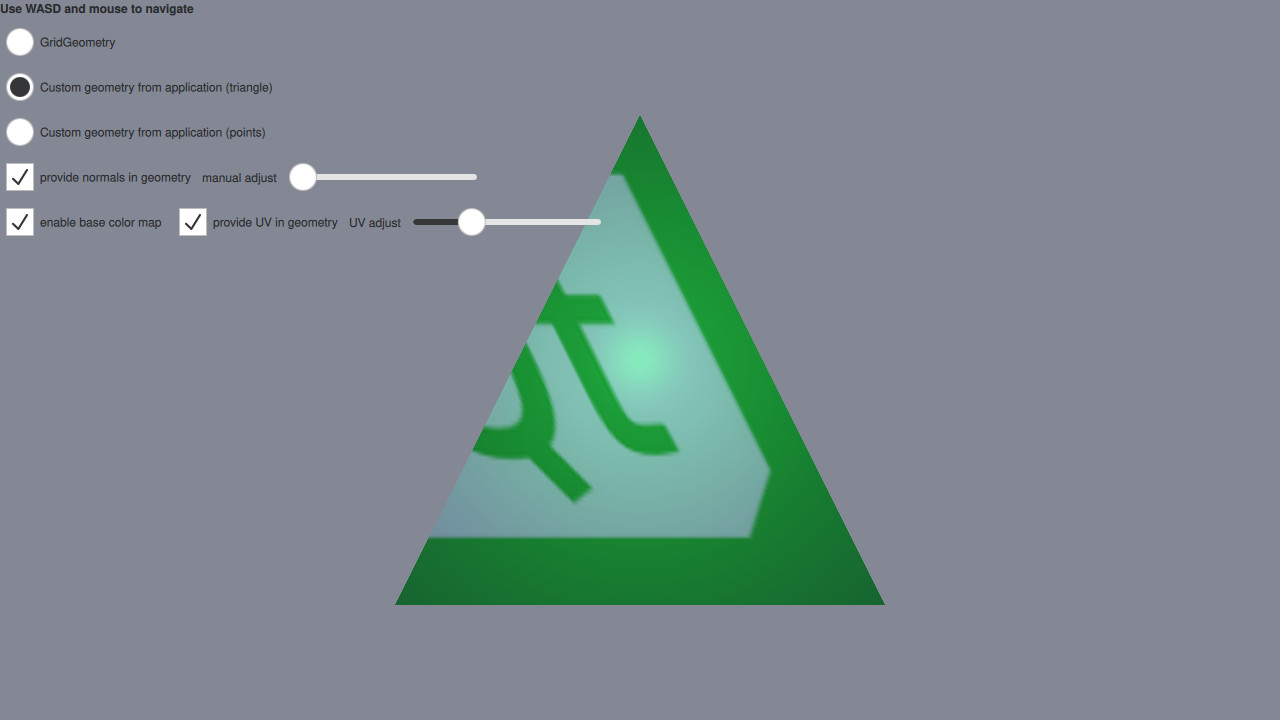# Qt Quick 3D - Custom Geometry Example

Demonstrates providing custom vertex data from C++.This example makes use of QQuick3DGeometry and the geometry property of Model to render a mesh with vertex, normal, and texture coordinates specified from C++ instead of a pre-baked asset.

In addition, the GridGeometry is also demonstrated. GridGeometry is a built-in QQuick3DGeometry implementation that provides a mesh with line primitives suitable for displaying a grid.

The focus on this example will be on the code that provides the custom geometry, so let's first have a look at the `ExampleTriangleGeometry` C++ header file:

```class ExampleTriangleGeometry : public QQuick3DGeometry
{
Q_OBJECT
QML_NAMED_ELEMENT(ExampleTriangleGeometry)
Q_PROPERTY(bool normals READ normals WRITE setNormals NOTIFY normalsChanged)
Q_PROPERTY(float normalXY READ normalXY WRITE setNormalXY NOTIFY normalXYChanged)
Q_PROPERTY(bool uv READ uv WRITE setUV NOTIFY uvChanged)

public:
ExampleTriangleGeometry();

bool normals() const { return m_hasNormals; }
void setNormals(bool enable);

float normalXY() const { return m_normalXY; }
void setNormalXY(float xy);

bool uv() const { return m_hasUV; }
void setUV(bool enable);

signals:
void normalsChanged();
void normalXYChanged();
void uvChanged();

private:
void updateData();

bool m_hasNormals = false;
float m_normalXY = 0.0f;
bool m_hasUV = false;
};```

The most important thing to notice is that our `ExampleTriangleGeometry` class inherits from QQuick3DGeometry and that we call the `QML_NAMED_ELEMENT(ExampleTriangleGeometry)` macro, making our class accessible in QML. There are also a few properties defined through the `Q_PROPERTY` macro which are automatically exposed in our QML object. Now, let's look at the QML Model:

```Model {
scale: Qt.vector3d(100, 100, 100)
geometry: ExampleTriangleGeometry {
normals: cbNorm.checked
normalXY: sliderNorm.value
uv: cbUV.checked
}
materials: [
DefaultMaterial {
Texture {
id: baseColorMap
source: "qt_logo_rect.png"
}
cullMode: DefaultMaterial.NoCulling
diffuseMap: cbTexture.checked ? baseColorMap : null
specularAmount: 0.5
}
]
}```

Note that we specify the `geometry` property to use our `ExampleTriangleGeometry` class, with the relevant properties specified. This is all that is needed on the QML side to use a custom geometry.

Now, lets look at the other important part of the C++ code, namely the `updateData()` method. This method creates and uploads the data for our custom geometry whenever a `ExampleTriangleGeometry` class is created or any of its QML properties are updated.

```void ExampleTriangleGeometry::updateData()
{
clear();

int stride = 3 * sizeof(float);
if (m_hasNormals)
stride += 3 * sizeof(float);
if (m_hasUV)
stride += 2 * sizeof(float);

QByteArray vertexData(3 * stride, Qt::Initialization::Uninitialized);
float *p = reinterpret_cast<float *>(vertexData.data());

// a triangle, front face = counter-clockwise
*p++ = -1.0f; *p++ = -1.0f; *p++ = 0.0f;
if (m_hasNormals) {
*p++ = m_normalXY; *p++ = m_normalXY; *p++ = 1.0f;
}
if (m_hasUV) {
}
*p++ = 1.0f; *p++ = -1.0f; *p++ = 0.0f;
if (m_hasNormals) {
*p++ = m_normalXY; *p++ = m_normalXY; *p++ = 1.0f;
}
if (m_hasUV) {
}
*p++ = 0.0f; *p++ = 1.0f; *p++ = 0.0f;
if (m_hasNormals) {
*p++ = m_normalXY; *p++ = m_normalXY; *p++ = 1.0f;
}
if (m_hasUV) {
}

setVertexData(vertexData);
setStride(stride);
setBounds(QVector3D(-1.0f, -1.0f, 0.0f), QVector3D(+1.0f, +1.0f, 0.0f));

setPrimitiveType(QQuick3DGeometry::PrimitiveType::Triangles);

0,
QQuick3DGeometry::Attribute::F32Type);

if (m_hasNormals) {
3 * sizeof(float),
QQuick3DGeometry::Attribute::F32Type);
}

if (m_hasUV) {
m_hasNormals ? 6 * sizeof(float) : 3 * sizeof(float),
QQuick3DGeometry::Attribute::F32Type);
}
}```

The method starts by calling `clear()` to clear all previously uploaded data. It then computes the stride for the vertices, taking into account the presence of normals and uv coordinates. Then a byte array is created to hold the vertex buffer, which is then filled with vertices for a single triangle with corners in (-1, -1, 0), (1, -1, 0) and (0, 1, 0).

Then the vertex data is uploaded and the stride is set by calling `setVertexData()` and `setStride()`. The bounds of the geometry is set by calling `setBounds`. Although not used in this example setting the bounds is needed for shadows to work. Then the primitive type is set by calling `setPrimitiveType()`. Lastly, we specify how the attributes for position, normal and uv coords are laid out in memory in the previously uploaded buffer by calling `addAttribute()` for each attribute.

Files:

Images: